### Home > INT3S > Chapter 7 > Lesson 7.2.5 > Problem7-116

7-116.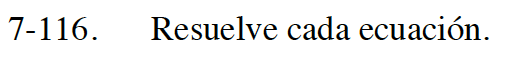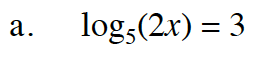53 = 2x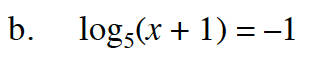5−1 = x + 1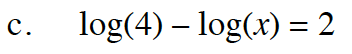$\log_{10} \left (\frac{4}{x} \right )=2$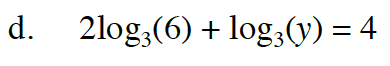log3(62) + log3(y) = 4